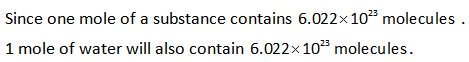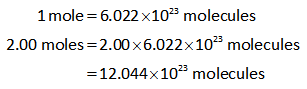# The mole is defined as the number of atoms in exactly 12 gg of carbon-12. The mole equals 6.022×10236.022×1023 items of whatever you are counting. This number is also called Avogadro's number, after Amadeo Avogadro (1776–1856).Molar mass is the mass of 1 molmol of atoms of an element; for compounds, molar mass is the mass of 1 molmol of molecules or formula units. Molar mass can be used to convert mass to moles for any element or compound.How many water molecules are in a block of ice containing 2.00 molmol of water (H2OH2O)?

Question
1 views

The mole is defined as the number of atoms in exactly 12 gg of carbon-12. The mole equals 6.022×10236.022×1023 items of whatever you are counting. This number is also called Avogadro's number, after Amadeo Avogadro (1776–1856).

Molar mass is the mass of 1 molmol of atoms of an element; for compounds, molar mass is the mass of 1 molmol of molecules or formula units. Molar mass can be used to convert mass to moles for any element or compound.

How many water molecules are in a block of ice containing 2.00 molmol of water (H2OH2O)?

check_circle

star
star
star
star
star
1 Rating
Introdcution

Avogadro number is the unit used for 1 mole of chemical substance. It is applied to convert the given moles into the particles or molecules. It helps to find out that how many particles/molecules are present in a mole of given substance.

CalculationHence the water molecules present in a block of ice having 2.00 moles of water can be calculated as shown below....

### Want to see the full answer?

See Solution

#### Want to see this answer and more?

Solutions are written by subject experts who are available 24/7. Questions are typically answered within 1 hour.*

See Solution
*Response times may vary by subject and question.
Tagged in